# Exploratory Data Analysis: Univariate, Bivariate, and Multivariate Analysis

## Introduction

The importance of data in machine learning is enormous. It lies at the heart of Machine Learning and Data Science techniques. Data is a raw material, and it needs to be analyzed thoroughly to know its quality. If it has high quality and is present in high volume, we can achieve better results even with simple machine learning algorithms. However, it is expensive to have both quality and quantity high. Every day, enterprises are collecting tons of data. But, deriving valuable patterns and insights from this data for making knowledgeable business decisions require knowledge of exploratory data analysis. In this session, we will look into some basic data analysis techniques based on the nature of the data and requirements.

Exploratory data analysis can be classified as Univariate, Bivariate, and Multivariate analysis. Let's explore each of these classifications in greater detail.

### Key takeaways from the blog

• What is the univariate analysis?
• What are the types of univariate analysis in machine learning?
• What is bivariate analysis?
• What are the types of bivariate analysis?
• What is multivariate analysis?
• What are the methods used for multivariate analysis?

## What is Univariate Analysis?

'Uni' refers to one, and 'variate' means variable, the word univariate refers to the analysis involving a single variable. The analysis can include summarization, measurements of dispersion, measurements of central tendency, and visualizations like histograms, distributions, frequency tables, bar charts, pie charts, boxplots, etc. The idea is simply that the data must contain a single variable, and it could be a categorical or numeric variable. Let's start our Univariate analysis by discussing some basic methods.

## What are the types of univariate analysis?

Let's dive deeper into the different types of analysis involved in the univariate analysis.

### Frequency distribution analysis

This analysis is used to analyze continuous numerical data where we try to extract the statistical summary of the feature.

• Maximum, minimum, and mean (average) analysis: Information like maximum, minimum, and mean values of any numerical data gives us a great impression of how that feature is distributed. Suppose we are analyzing the age of our customers. We saw that the minimum age of our customers is 18, the maximum age is 26, and the average age is 22. We can extract information that our customers are youth.
• Standard deviation and variance analysis: We have the mean value from the earlier step. To analyze each sample present in the data, we can take the reference of the mean and calculate the deviation of that sample from it. This is known as standard deviation and is used to estimate the dispersion present in the data. High dispersion means samples are widespread, and low dispersion means samples are very close to the mean value.

### Histograms

A histogram plots the distribution of a numeric variable as a sequence of bars. Each bar in a histogram covers a range of values called bins. The "total range" of the dataset is divided into a number of equal parts, which are known as bins or class intervals. There's no defined way for finding the bins, but generally, we avoid using too many and too few bins. Also, changing the bin size changes the histogram. The height of the histogram represents the frequency of values falling within the corresponding bin. Let's implement a histogram to visualize the univariate data:

``````import seaborn as sns
sns.histplot(data=penguins['flipper_length_mm'], kde=True);
``````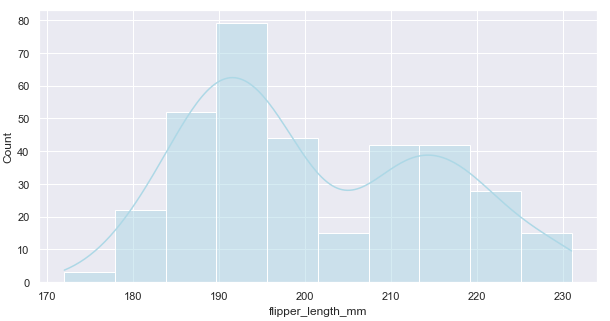The above histogram displays the distribution of Penguin's flipper_length in millimeters. Here, the bin values can be confirmed using the below line.

``````np.histogram(penguins['flipper_length_mm'].dropna())
``````

The majority of the Penguin's flipper length is between 183 to 195mm.

Histograms are perfect for exhibiting the general distribution of features. We can tell whether the distribution is symmetric or skewed (unsymmetric) using the histogram. Additionally, we can comment on the presence of outliers. Please refer to this blog if unfamiliar with the symmetric and skewed distributions.

### Pie Charts

A Pie Chart is a visualization of univariate data that depicts the data in a circular diagram. Each slice of the pie chart corresponds to a relative proportion of the category versus the entire group. In other words, the parts/slice of the graph is proportionate to the fraction of the whole in each category. The pie chart comprises 100% of all categories, while the piece represents the categories within the data.

``````import seaborn as sns
import matplotlib.pyplot as plt

fig, ax = plt.subplots()
labels = ['Ocean', 'Land']

percentages = [70.8, 29.2]
explode=(0.1,0)
ax.pie(percentages, explode=explode, labels=labels,
colors=color_palette_list[0:2], autopct='%1.0f%%',
pctdistance=1.2,labeldistance=1.4)

ax.axis('equal')
ax.set_title("Land to Ocean Ratio")
ax.legend(bbox_to_anchor=(1, 1));
``````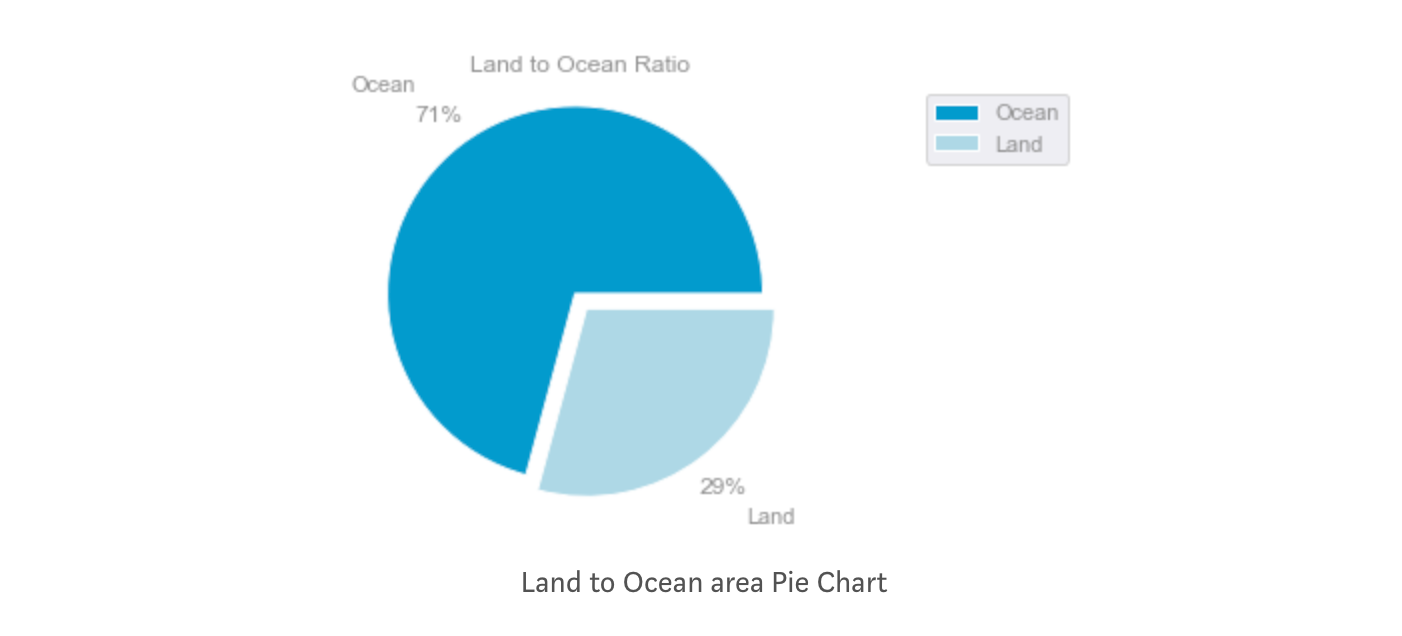The above pie chart shows the percentage of earth captured by land and water. As per the pie chart, 29% of the earth is captured by land while 71% is covered with water. Informative and straightforward.

### Boxplot

A boxplot or whisker plot is a diagram often used for visualizing the distribution of numeric values. A boxplot divides the data into equal parts using the three quartiles, and this serves as an excellent visualization of distribution. A boxplot consists of the lowest value, the first quartile (Lower Quartile), the Second quartile (Median), the Third quartile (Upper Quartile), and finally, the highest value. A quartile is a statistical term used to describe the division of observations. The mentioned three quartiles divide the data into four equal parts. This can be confirmed using the illustration given below: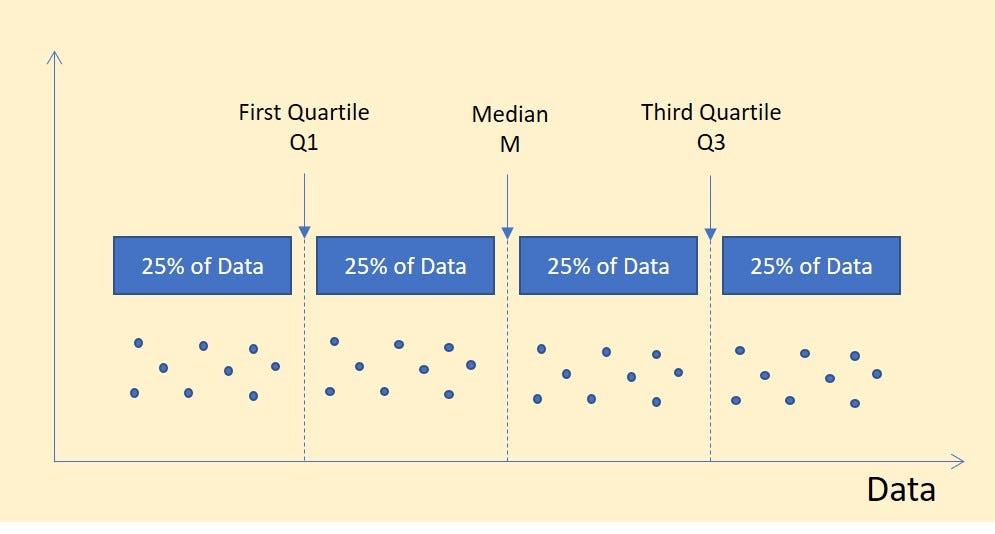Let's implement a boxplot:

``````x = np.random.normal(0, 1, 10000)
mean = x.mean()
std = x.std()
q1, median, q3 = np.percentile(x, [25, 50, 75])
iqr = q3 - q1

fig, (ax1, ax2) = plt.subplots(nrows=2, sharex=True, figsize=(13,8))

medianprops = dict(linestyle='-', linewidth=2, color='yellow')
sns.boxplot(x=x, color='#009ACD', saturation=1, medianprops=medianprops,
flierprops={'markerfacecolor': 'mediumseagreen'}, whis=1.5, ax=ax1)
``````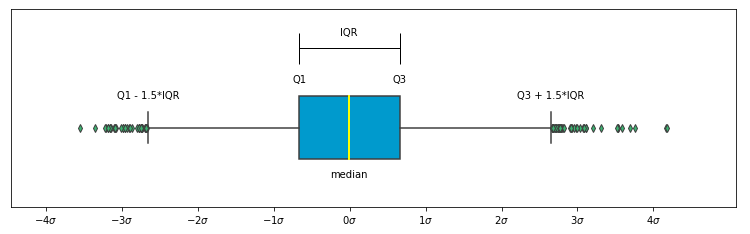The above box plot is generated from a normal distribution, and because of that, it is approximately symmetric with respect to the middle yellow line.

The Inter Quartile Range (IQR) represents the middle 50% values. Each quartile to end or quartile covers 25% of the data. Hence, IQR is the difference between the third and the first quartile.

IQR = (Third Quartile (Q3)- First Quartile (Q1))

IQR can be used to find the outliers in the data. A detailed approach has been discussed in this blog

Boxplot can help in visualizing the distribution of data. The image below can distinguish the skewed distributions vs. the normal distribution pattern.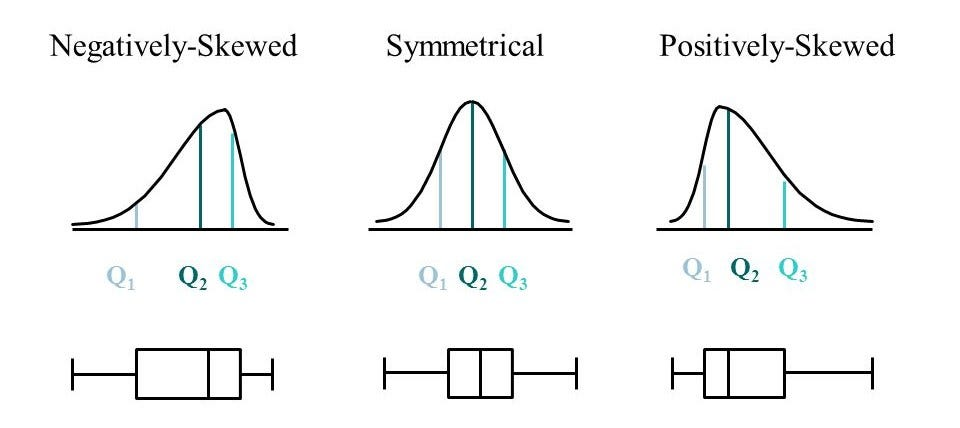### Bar Chart

A bar chart plots the count of categories within a feature as bars. It is only applicable to the categorical data. The category level is mentioned over the x-axis, while the frequency of the categories is mentioned over the y-axis. Each category in the feature will have a corresponding bar value stating the frequency of class appearing in the feature. Also, the bars are plotted on a baseline for easy comparison. Let's implement a bar chart:

``````import matplotlib.pyplot as plt
fig = plt.figure(figsize=(8, 5))

langs = ['Math', 'Science', 'Economics', 'Health Education', 'English']

students = [16, 13, 15, 9, 6]
ax.set_title("Subjects taken by Number of Students", fontsize = 15)

plt.xlabel("Subjects", fontsize = 14)
plt.ylabel("Number of Students", fontsize = 14)
plt.show()
``````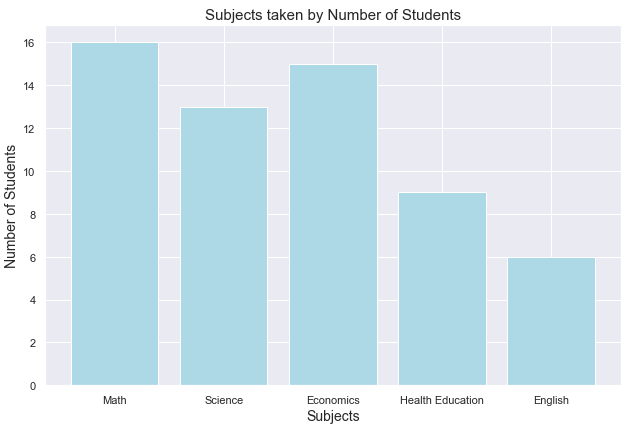## What is Bivariate Analysis?

'Bi' means two, and 'variate' means variable. Collectively, Bivariate analysis refers to the exploratory data analysis between two variables. Now again, the variables can be either numeric or categorical. Bivariate analysis helps in studying the relationship between two variables, and if the two variables are related, we can comment on the strength of association. Let's discuss and implement some basic bivariate EDA techniques:

## What are the types of bivariate analysis?

We know the types of data can be either numerical or categorical. So there can be three types of scenarios:

• Numerical feature vs. Numerical feature
• Categorical feature vs. Categorical feature
• Numerical feature vs. Categorical feature

Let's look at some methods to do the bivariate analysis.

### Scatter Plot (Numeric vs. Numeric)

A scatter plot or scatter graph is used to plot data points corresponding to two features. This helps explain the change in one variable with respect to the change in the other one. A dot in the scatterplot represents each row of the dataset. This also helps explain the correlation between two variables, but primarily, scatter plots are used to establish the relationship between two variables.

``````iris = sns.load_dataset('iris')
sns.scatterplot(data=iris, x='sepal_length', y='petal_length', hue='species')
plt.xlabel('Sepal Length')
plt.ylabel('Petal Length')
plt.show()
``````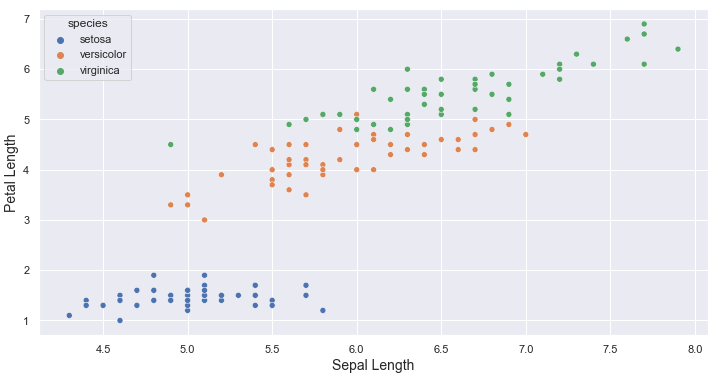The above scatterplot clearly shows the presence of three distinct clusters of different flower species. On the X-axis, we have the Sepal length of the flower, while on the Y-axis, we have the Petal length. The scatterplot indicates a strong positive correlation between Sepal Length and Petal Length.

How can we comment on the correlation just by looking at the scatterplot? The image below will illustrate how we can comment on the correlation between two variables by looking at the scatterplot.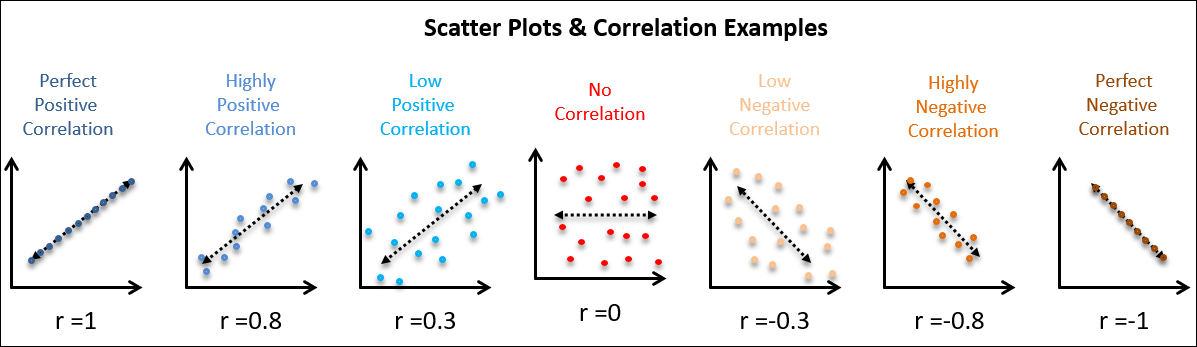Correlation varies between -1 to 1. A correlation of positive one indicates a perfect positive linear relationship, while a negative one indicates a perfectly inverse relationship between two variables. Further, a correlation of zero indicates no connection between the two variables.

### Chi-Squared Test(Categorical vs. Categorical)

Chi-Squared Test is used to describe the relationship between categorical variables. It is a hypothesis test developed to test the statistical significance of the relationship between two categorical variables. It tells us whether the two variables are related or not. It works by calculating the Chi Statistics, which is calculated using the below formula:Here, O represents the Observed Values, and E represents the Expected Values. This Chi Statistics is calculated and compared with the critical Chi value corresponding to the degrees of freedom (c) and decided significance level. In statistics, the degrees of freedom (c) indicate the number of independent values that can alter an analysis without breaking any restrictions. Finally, a Null Hypothesis is tested against an alternate hypothesis which is either rejected or accepted based on the difference between chi statistics and critical chi value. Please follow this blog if you're not aware of null hypothesis testing.

### Analysis of Variance: ANOVA (Continuous vs. Categorical)

ANOVA is a statistical test used to describe the potential differences in a continuous dependent variable by a categorical (Nominal) variable having two or more classes. It splits the observed variability in the data into two parts:

• Systematic Factors
• Random Factors

Systematic Factors have a statistically significant influence on the data, while the random factors don't add any information. ANOVA can explain the impact of an independent variable over the dependent variable. When there's only one dependent variable and one independent variable, it is known as one-way ANOVA.

For instance, suppose we want to find the influence of weekdays over the parameter hotel price. Naturally, the hotel's price might be lower on weekdays to attract the crowd. Alternatively, on weekends, hotel prices rise because demand rises. Let's confirm if the day of the week influences the hotel prices.

``````import pandas as pd
import numpy as np
import statsmodels.api as sm
from statsmodels.formula.api import ols

df = pd.DataFrame({'weekday': np.repeat(['Weekday', 'Weekend'], 10),
'hotel_price': [96, 94, 89, 105, 110, 100, 102, 98, 91, 104, 122, 114, 119, 115, 122, 109, 111, 106, 107, 113]})

model = ols('hotel_price ~ C(weekday)', data=df).fit()
sm.stats.anova_lm(model, typ=1)
``````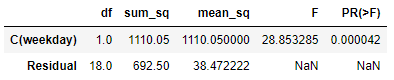Now, the P-value for weekdays is 0.000042, which is less than 0.05, which means weekday is highly significant in determining Hotel Price. ANOVA's result tells us that hotel prices are highly influenced by the day of the week, which is intuitively true.

## What is Multivariate Analysis?

'Multi' means many, and 'variate' means variable. Multivariate analysis refers to the statistical procedure for analyzing the data involving more than two variables. Alternatively, this can be used to analyze the relationship between dependent and independent variables. Multivariate analysis has various applications in clustering, feature selection, root-cause analysis, hypothesis testing, dimensionality reduction, etc.

## What are the methods used for multivariate analysis?

We can easily correlate the multivariate with the unsupervised learning techniques in machine learning. Unsupervised learning techniques are used to analyze patterns present in the data. The popular methods associated with it are clustering and dimensionality reduction. Let's have a look at these techniques.

### Clustering Analysis

Clustering analysis segregates the data points into groups known as clusters. The data is grouped into clusters based on the similarity between the multivariate features. This data mining technique allows us to understand the data distribution based on the available features. Let's implement the K-means clustering algorithm over the Iris dataset:

For the demonstration, we will remove the species column and find the optimum number of clusters using the elbow plot. Here's a link if you are not familiar with the k-means algorithm. Remember, our goal is to group similar data points in a cluster, but we need to find the optimum clusters before that. Let's apply the elbow technique:

``````iris = sns.load_dataset("iris")
iris.drop(['species'], axis=1, inplace=True)
normalizer = MinMaxScaler().fit(iris)
iris = normalizer.transform(iris)

distortions = []
inertias = []
K = range(1, 10)

for k in K:
kmeans = KMeans(n_clusters=k).fit(iris)
kmeans.fit(iris)

distortions.append(sum(np.min(cdist(iris, kmeans.cluster_centers_,'euclidean'), axis=1)) / iris.shape)
inertias.append(kmeans.inertia_)

plt.plot(K, distortions, 'bx-')
plt.xlabel('Number of Clusters', fontsize = 13)
plt.ylabel('Distortion or SSE', fontsize = 13)
plt.title('SSE vs Number of Clusters - Elbow Plot', fontsize = 13)
plt.show()
``````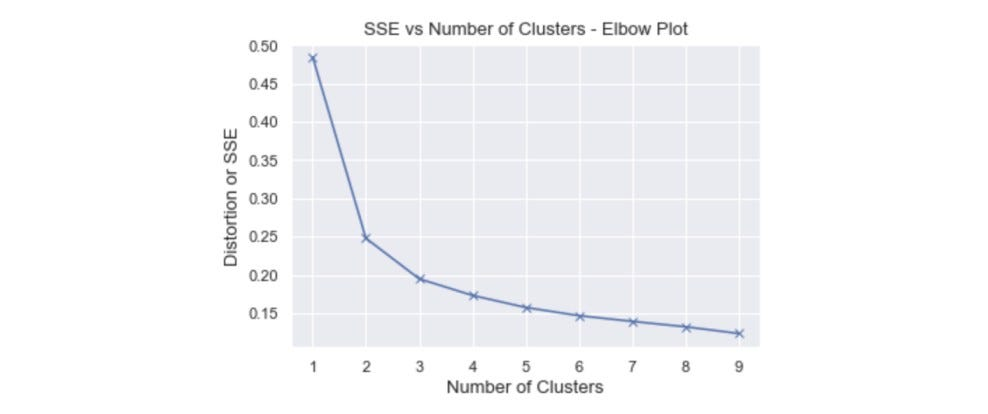The Elbow appears at k = 3, and hence, it will be the optimum number of clusters for the K-means algorithm.

``````kmeans = KMeans(n_clusters=3)
kmeans.fit(iris)

iris['clusters'] = kmeans.fit_predict(iris)
iris.columns = ['sepal_length', 'sepal_width', 'petal_length', 'petal_width', 'clusters']

plt.scatter(iris['sepal_length'],iris['petal_length'],c=iris["clusters"],cmap='rainbow')
plt.xlabel("Sepal Length", fontsize=14)
plt.ylabel("Petal Length", fontsize=14)
plt.show();
``````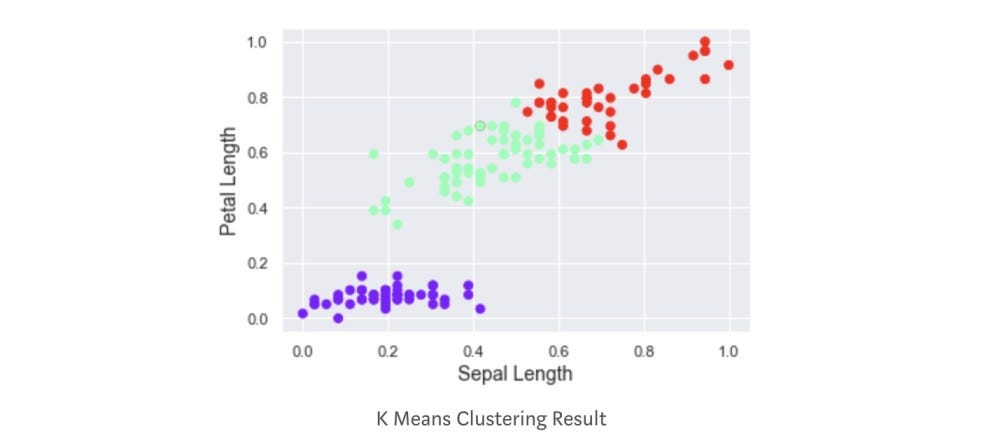From the above plot, we can visualize the three clusters. We have successfully grouped similar data points.

### Principal Component Analysis (PCA)

PCA is a dimensionality reduction technique frequently used to reduce the dimensions of large datasets that exhibit multicollinearity. In PCA, the original data is transformed into a new set of features such that a fewer number of transformed features explains the variance of the original dataset. This comes at a minimal loss of information. For a deep understanding of PCA, visit this blog.

Let's implement PCA on the credit card dataset:

``````import pandas as pd
import numpy as np
import matplotlib.pyplot as plt
from sklearn.decomposition import PCA

transaction_data.drop("Time", axis=1, inplace=True)
transaction_feature = transaction_data.iloc[:,:-2]

``````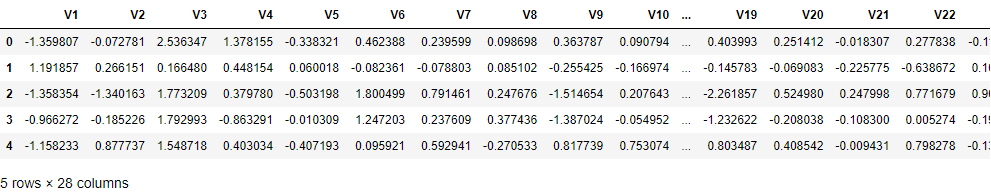This dataset contains 28 features, and we aim to reduce the number of features.

``````pca = PCA()
transaction_feature = pca.fit_transform(transaction_feature)
explained_variance = pca.explained_variance_ratio_

print(explained_variance*100)
``````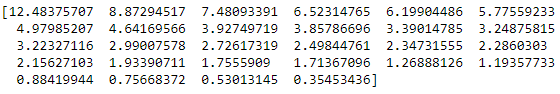Initial 17 principal components contribute to 85% variance of the original data. Let's also visualize this using the Scree plot:

``````PC_values = np.arange(pca.n_components_) + 1
plt.plot(PC_values, pca.explained_variance_ratio_, 'o-', linewidth=2, color='blue')
plt.axhline(y=0.023, color='r', linestyle=' - ')
plt.title('Scree Plot', fontsize=15)
plt.xlabel('Principal Component', fontsize=14)
plt.ylabel('Variance Explained', fontsize=14)
plt.show()
``````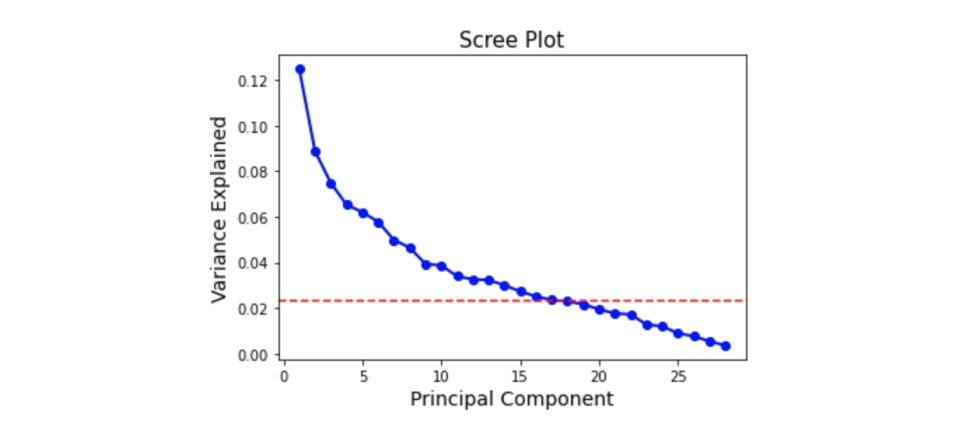``````pca = PCA(n_components=17)
reduced_features = pca.fit_transform(transaction_feature)

reduced_features = pd.DataFrame(reduced_features)
``````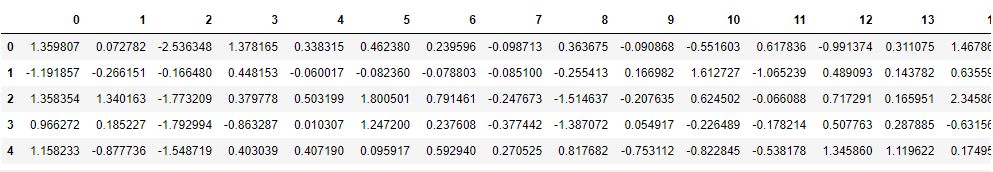``````reduced_features.shape

## (284807, 17)
``````

Finally, we have only 17 features in the final dataset at the cost of a 15% variance loss.

### Multiple Correspondance Analysis (MCA)

Correspondence Analysis is a powerful data visualization technique frequently utilized for visualizing the relationship between categories. This is applicable when data is multinomial categorical and highly used in surveys and questionnaires for association mining.

MCA works by separating the respondents based on their categories. For instance, respondents or individuals falling into the same categories are plotted next to each other, while respondents in different categories are plotted as far as possible. This will form a cluster of similar respondents or individuals, which can be visualized in a plot. Also, this is a distance-based approach.

#### Advantages of using Multiple Correspondance Analysis (MCA)

• Explains how categorical features are associated with each other.
• Explains whether individuals or respondents shares similarity with the categorical variables.
• Provides visualization explaining the association between categories.

#### When do we use MCA?

• When there are no missing values or negative values in the dataset.
• All the data has the same scale.
• Data must contain at least two columns.
• When the dataset contains categorical features.

Let's implement Multiple Correspondance Analysis:

``````import pandas as pd
import prince
import numpy as np

``````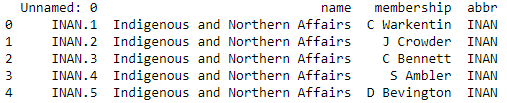``````mca = prince.MCA()
mca_data = mca.fit(X)
mca_X = mca_data.transform(X)

ax = mca.plot_coordinates(
X=X,
ax=None,
figsize=(6, 6),
show_row_points=True,
row_points_size=10,
show_row_labels=False,
show_column_points=True,
column_points_size=30,
show_column_labels=False,
legend_n_cols=1)
``````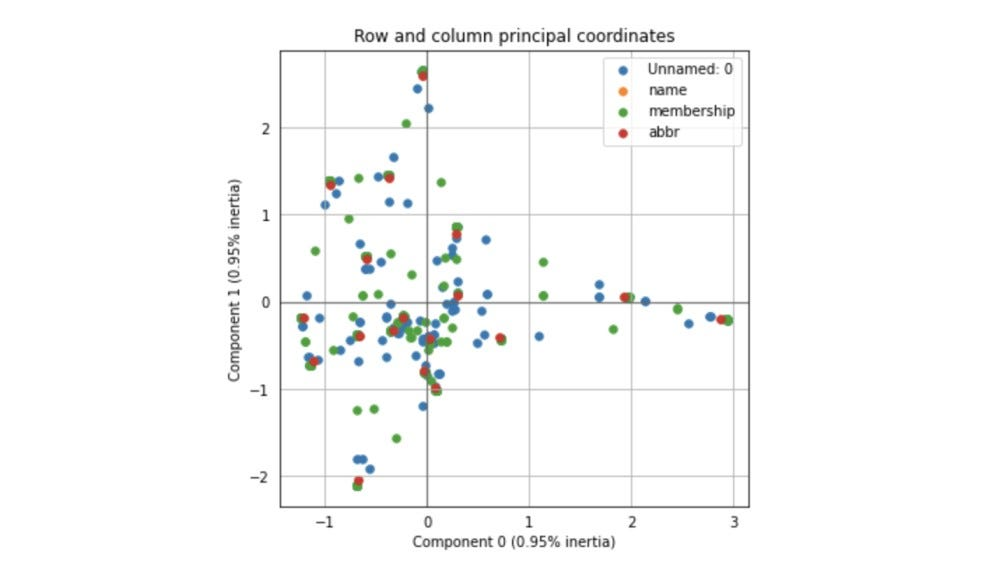## Possible Interview Questions

These are some popular questions asked on this topic:

• What is the difference between univariate, bivariate, and multivariate analysis?
• What are the types of univariate, bivariate, and multivariate analysis?
• Explain the ANOVA technique and the category for which it is used.
• Explain Multiple Correspondance Analysis (MCA).
• How does the correlation between features represent the relationship between two features?

## Conclusion

In this session, we briefly discussed the different methods used for data analysis, namely the Univariate, Bivariate, and Multivariate analysis techniques. These are classified based on the number of variables involved in the analysis. Under each analysis, we discussed some methods used to analyze the data and implemented them in python under each analysis. Choosing the correct way for the analysis depends on the type of data we are handling and the number of variables involved in the analysis. We haven't covered more strategies in this session, but knowing the above techniques is essential for any data analyst.

Enjoy learning, Enjoy algorithms!